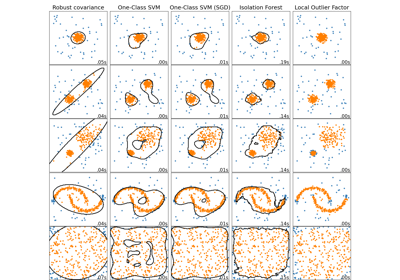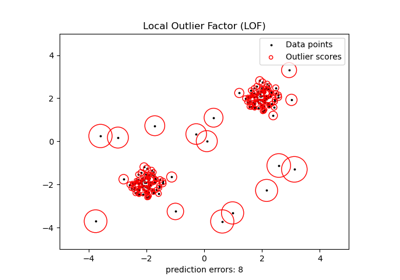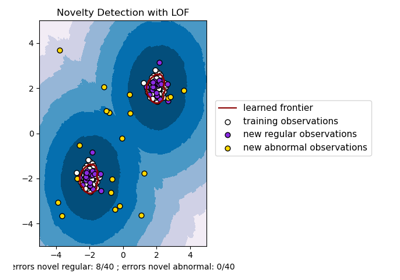# sklearn.neighbors.LocalOutlierFactor¶

class sklearn.neighbors.LocalOutlierFactor(n_neighbors=20, algorithm=’auto’, leaf_size=30, metric=’minkowski’, p=2, metric_params=None, contamination=’legacy’, novelty=False, n_jobs=None)[source]

Unsupervised Outlier Detection using Local Outlier Factor (LOF)

The anomaly score of each sample is called Local Outlier Factor. It measures the local deviation of density of a given sample with respect to its neighbors. It is local in that the anomaly score depends on how isolated the object is with respect to the surrounding neighborhood. More precisely, locality is given by k-nearest neighbors, whose distance is used to estimate the local density. By comparing the local density of a sample to the local densities of its neighbors, one can identify samples that have a substantially lower density than their neighbors. These are considered outliers.

Parameters: n_neighbors : int, optional (default=20) Number of neighbors to use by default for kneighbors queries. If n_neighbors is larger than the number of samples provided, all samples will be used. algorithm : {‘auto’, ‘ball_tree’, ‘kd_tree’, ‘brute’}, optional Algorithm used to compute the nearest neighbors: ‘ball_tree’ will use BallTree ‘kd_tree’ will use KDTree ‘brute’ will use a brute-force search. ‘auto’ will attempt to decide the most appropriate algorithm based on the values passed to fit method. Note: fitting on sparse input will override the setting of this parameter, using brute force. leaf_size : int, optional (default=30) Leaf size passed to BallTree or KDTree. This can affect the speed of the construction and query, as well as the memory required to store the tree. The optimal value depends on the nature of the problem. metric : string or callable, default ‘minkowski’ metric used for the distance computation. Any metric from scikit-learn or scipy.spatial.distance can be used. If ‘precomputed’, the training input X is expected to be a distance matrix. If metric is a callable function, it is called on each pair of instances (rows) and the resulting value recorded. The callable should take two arrays as input and return one value indicating the distance between them. This works for Scipy’s metrics, but is less efficient than passing the metric name as a string. Valid values for metric are: from scikit-learn: [‘cityblock’, ‘cosine’, ‘euclidean’, ‘l1’, ‘l2’, ‘manhattan’] from scipy.spatial.distance: [‘braycurtis’, ‘canberra’, ‘chebyshev’, ‘correlation’, ‘dice’, ‘hamming’, ‘jaccard’, ‘kulsinski’, ‘mahalanobis’, ‘minkowski’, ‘rogerstanimoto’, ‘russellrao’, ‘seuclidean’, ‘sokalmichener’, ‘sokalsneath’, ‘sqeuclidean’, ‘yule’] See the documentation for scipy.spatial.distance for details on these metrics: https://docs.scipy.org/doc/scipy/reference/spatial.distance.html p : integer, optional (default=2) Parameter for the Minkowski metric from sklearn.metrics.pairwise.pairwise_distances. When p = 1, this is equivalent to using manhattan_distance (l1), and euclidean_distance (l2) for p = 2. For arbitrary p, minkowski_distance (l_p) is used. metric_params : dict, optional (default=None) Additional keyword arguments for the metric function. contamination : float in (0., 0.5), optional (default=0.1) The amount of contamination of the data set, i.e. the proportion of outliers in the data set. When fitting this is used to define the threshold on the decision function. If “auto”, the decision function threshold is determined as in the original paper. Changed in version 0.20: The default value of contamination will change from 0.1 in 0.20 to 'auto' in 0.22. novelty : boolean, default False By default, LocalOutlierFactor is only meant to be used for outlier detection (novelty=False). Set novelty to True if you want to use LocalOutlierFactor for novelty detection. In this case be aware that that you should only use predict, decision_function and score_samples on new unseen data and not on the training set. n_jobs : int or None, optional (default=None) The number of parallel jobs to run for neighbors search. None means 1 unless in a joblib.parallel_backend context. -1 means using all processors. See Glossary for more details. Affects only kneighbors and kneighbors_graph methods. negative_outlier_factor_ : numpy array, shape (n_samples,) The opposite LOF of the training samples. The higher, the more normal. Inliers tend to have a LOF score close to 1 (negative_outlier_factor_ close to -1), while outliers tend to have a larger LOF score. The local outlier factor (LOF) of a sample captures its supposed ‘degree of abnormality’. It is the average of the ratio of the local reachability density of a sample and those of its k-nearest neighbors. n_neighbors_ : integer The actual number of neighbors used for kneighbors queries. offset_ : float Offset used to obtain binary labels from the raw scores. Observations having a negative_outlier_factor smaller than offset_ are detected as abnormal. The offset is set to -1.5 (inliers score around -1), except when a contamination parameter different than “auto” is provided. In that case, the offset is defined in such a way we obtain the expected number of outliers in training.

References

 [Rca479bb49841-1] Breunig, M. M., Kriegel, H. P., Ng, R. T., & Sander, J. (2000, May). LOF: identifying density-based local outliers. In ACM sigmod record.

Methods

 fit(self, X[, y]) Fit the model using X as training data. get_params(self[, deep]) Get parameters for this estimator. kneighbors(self[, X, n_neighbors, …]) Finds the K-neighbors of a point. kneighbors_graph(self[, X, n_neighbors, mode]) Computes the (weighted) graph of k-Neighbors for points in X set_params(self, \*\*params) Set the parameters of this estimator.
__init__(self, n_neighbors=20, algorithm=’auto’, leaf_size=30, metric=’minkowski’, p=2, metric_params=None, contamination=’legacy’, novelty=False, n_jobs=None)[source]
decision_function

Shifted opposite of the Local Outlier Factor of X.

Bigger is better, i.e. large values correspond to inliers.

The shift offset allows a zero threshold for being an outlier. Only available for novelty detection (when novelty is set to True). The argument X is supposed to contain new data: if X contains a point from training, it considers the later in its own neighborhood. Also, the samples in X are not considered in the neighborhood of any point.

Parameters: X : array-like, shape (n_samples, n_features) The query sample or samples to compute the Local Outlier Factor w.r.t. the training samples. shifted_opposite_lof_scores : array, shape (n_samples,) The shifted opposite of the Local Outlier Factor of each input samples. The lower, the more abnormal. Negative scores represent outliers, positive scores represent inliers.
fit(self, X, y=None)[source]

Fit the model using X as training data.

Parameters: X : {array-like, sparse matrix, BallTree, KDTree} Training data. If array or matrix, shape [n_samples, n_features], or [n_samples, n_samples] if metric=’precomputed’. y : Ignored not used, present for API consistency by convention. self : object
fit_predict

“Fits the model to the training set X and returns the labels.

Label is 1 for an inlier and -1 for an outlier according to the LOF score and the contamination parameter.

Parameters: X : array-like, shape (n_samples, n_features), default=None The query sample or samples to compute the Local Outlier Factor w.r.t. to the training samples. y : Ignored not used, present for API consistency by convention. is_inlier : array, shape (n_samples,) Returns -1 for anomalies/outliers and 1 for inliers.
get_params(self, deep=True)[source]

Get parameters for this estimator.

Parameters: deep : boolean, optional If True, will return the parameters for this estimator and contained subobjects that are estimators. params : mapping of string to any Parameter names mapped to their values.
kneighbors(self, X=None, n_neighbors=None, return_distance=True)[source]

Finds the K-neighbors of a point. Returns indices of and distances to the neighbors of each point.

Parameters: X : array-like, shape (n_query, n_features), or (n_query, n_indexed) if metric == ‘precomputed’ The query point or points. If not provided, neighbors of each indexed point are returned. In this case, the query point is not considered its own neighbor. n_neighbors : int Number of neighbors to get (default is the value passed to the constructor). return_distance : boolean, optional. Defaults to True. If False, distances will not be returned dist : array Array representing the lengths to points, only present if return_distance=True ind : array Indices of the nearest points in the population matrix.

Examples

In the following example, we construct a NeighborsClassifier class from an array representing our data set and ask who’s the closest point to [1,1,1]

>>> samples = [[0., 0., 0.], [0., .5, 0.], [1., 1., .5]]
>>> from sklearn.neighbors import NearestNeighbors
>>> neigh = NearestNeighbors(n_neighbors=1)
>>> neigh.fit(samples)
NearestNeighbors(algorithm='auto', leaf_size=30, ...)
>>> print(neigh.kneighbors([[1., 1., 1.]]))
(array([[0.5]]), array([]))


As you can see, it returns [[0.5]], and [], which means that the element is at distance 0.5 and is the third element of samples (indexes start at 0). You can also query for multiple points:

>>> X = [[0., 1., 0.], [1., 0., 1.]]
>>> neigh.kneighbors(X, return_distance=False)
array([,
]...)

kneighbors_graph(self, X=None, n_neighbors=None, mode=’connectivity’)[source]

Computes the (weighted) graph of k-Neighbors for points in X

Parameters: X : array-like, shape (n_query, n_features), or (n_query, n_indexed) if metric == ‘precomputed’ The query point or points. If not provided, neighbors of each indexed point are returned. In this case, the query point is not considered its own neighbor. n_neighbors : int Number of neighbors for each sample. (default is value passed to the constructor). mode : {‘connectivity’, ‘distance’}, optional Type of returned matrix: ‘connectivity’ will return the connectivity matrix with ones and zeros, in ‘distance’ the edges are Euclidean distance between points. A : sparse matrix in CSR format, shape = [n_samples, n_samples_fit] n_samples_fit is the number of samples in the fitted data A[i, j] is assigned the weight of edge that connects i to j.

Examples

>>> X = [, , ]
>>> from sklearn.neighbors import NearestNeighbors
>>> neigh = NearestNeighbors(n_neighbors=2)
>>> neigh.fit(X)
NearestNeighbors(algorithm='auto', leaf_size=30, ...)
>>> A = neigh.kneighbors_graph(X)
>>> A.toarray()
array([[1., 0., 1.],
[0., 1., 1.],
[1., 0., 1.]])

predict

Predict the labels (1 inlier, -1 outlier) of X according to LOF.

This method allows to generalize prediction to new observations (not in the training set). Only available for novelty detection (when novelty is set to True).

Parameters: X : array-like, shape (n_samples, n_features) The query sample or samples to compute the Local Outlier Factor w.r.t. to the training samples. is_inlier : array, shape (n_samples,) Returns -1 for anomalies/outliers and +1 for inliers.
score_samples

Opposite of the Local Outlier Factor of X.

It is the opposite as bigger is better, i.e. large values correspond to inliers.

Only available for novelty detection (when novelty is set to True). The argument X is supposed to contain new data: if X contains a point from training, it considers the later in its own neighborhood. Also, the samples in X are not considered in the neighborhood of any point. The score_samples on training data is available by considering the the negative_outlier_factor_ attribute.

Parameters: X : array-like, shape (n_samples, n_features) The query sample or samples to compute the Local Outlier Factor w.r.t. the training samples. opposite_lof_scores : array, shape (n_samples,) The opposite of the Local Outlier Factor of each input samples. The lower, the more abnormal.
set_params(self, **params)[source]

Set the parameters of this estimator.

The method works on simple estimators as well as on nested objects (such as pipelines). The latter have parameters of the form <component>__<parameter> so that it’s possible to update each component of a nested object.

Returns: self

## Examples using sklearn.neighbors.LocalOutlierFactor¶Comparing anomaly detection algorithms for outlier detection on toy datasetsOutlier detection with Local Outlier Factor (LOF)Novelty detection with Local Outlier Factor (LOF)